# Examples

## Scalar FPT problem

Consider a time-homogeneous diffusion process with dynamics governed by the SDE: $dX_t = 0.5X_t(1-X_t^2)dt+0.5dB_t.$ Suppose we are interested in the first exit time of the process $$X_t$$ from the strip $$[-0.5,2]$$. we can then calculate the survival probability surface for all initial values on the strip $$[-0.5,2]$$ by solving a backward PDE (Tuckwell and Wan 1984). In R this can be achieved using the method of lines by way of the MOL.passage() function:

library("DiffusionRimp")

mu  <- function(X){(0.5*X*(1-X^2))}
sig <- function(X) {0.5}
res <- MOL.passage(Xs = 0.5,t = 10, limits=c(-0.5, 2), N = 51, delt = 1/250)

persp(res$Xt, res$time, res$surface, col = 'white', xlab = 'X_s', ylab = 'Time (t)', zlab = 'Survival Prob.', border = NA, shade = 0.5, theta = 145, phi = 30, ticktype = 'detailed')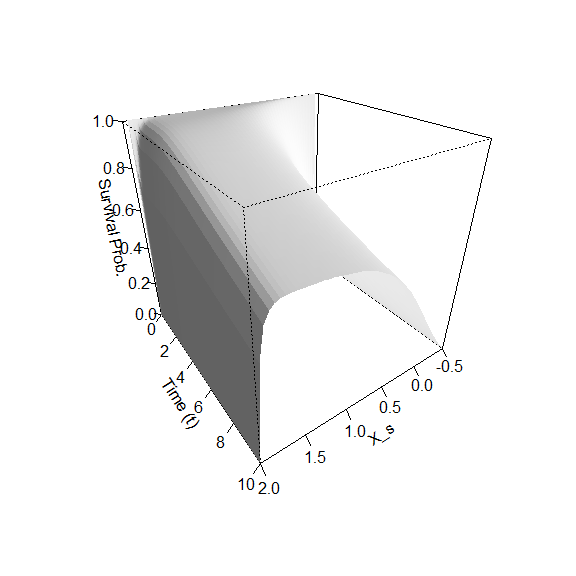plot(res$density~restime, col = '#222299', type='l', main = 'First passage time density')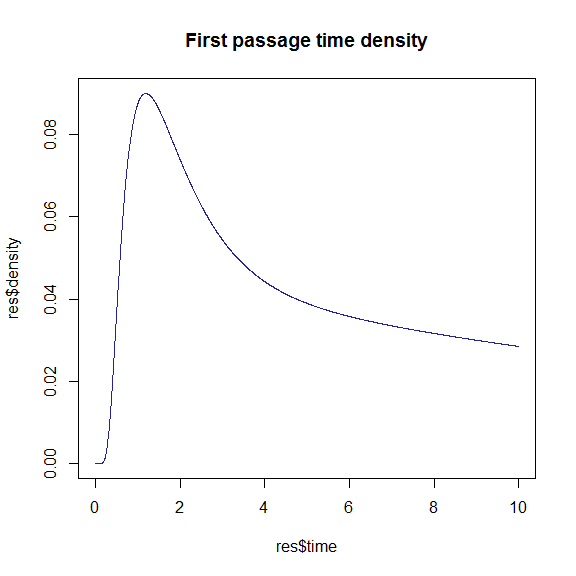#------------------------------------------------------------------------------- By supplying MOL.passage() with an initial value, the function will calculate a numerical derivative of the survival probability surface in order to calculate the first passage time density function. ## Bivariate FPT problem In similar fashion, we can calculate the survival probability surface for a bivariate diffusion escaping from an enclosed region. Consider a bivariate diffusion \begin{aligned} dX_t &=[0.5X_t(1 -X_t^2)+Y_t]dt + dB_t^{(1)}\\ dY_t &=[0.5Y_t(1 -Y_t^2)-X_t]dt + dB_t^{(2)}\\ \end{aligned} on the square region $$[-2, 2]\times [-2, 2]$$. Then we can calculate the evolution of the survival probability surface in R using: #=============================================================================== # Bi-cubic diffusion with concentration in the even quadrants. #=============================================================================== # Define drift and diffusion terms. mu1 <- function(X,Y){0.5*(1 - X^2)*X - Y} mu2 <- function(X,Y){0.5*(1 - Y^2)*Y - X} sig11 <- function(X,Y){1} sig22 <- function(X,Y){1} # Parameters of the problem. Xs <- 0.5 # Starting X-coordinate Ys <- 0.5 # Starting Y-coordinate t <- 10 # Final horizon time Xbar <- c(-2, 2) # Limits in X-dim Ybar <- c(-2, 2) # Limits in Y-dim Nodes <- 51 # How many nodes x nodes (incl. ends) delt <- 1/250 # Time step size res <- BiMOL.passage(Xs, Ys, t, c(Xbar, Ybar), Nodes, delt) time.sequence <- c(0.5, 2, 4, 6, 8, 10) k = 0 for(i in time.sequence) { k = k+1 persp(resXt, res$Yt, res$surface[,,i/delt], col='white',
xlab = 'State (X_0)', ylab = 'State (Y_0)',
zlab = 'Survival Probability', border = NA,
shade = 0.5, theta = 145 + 180*k/6)
}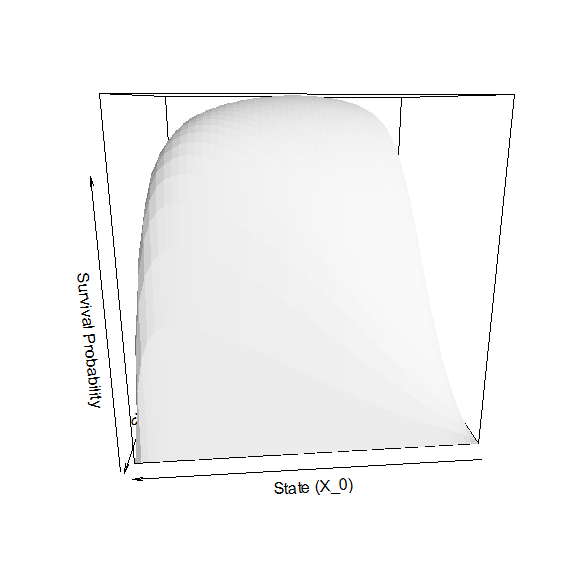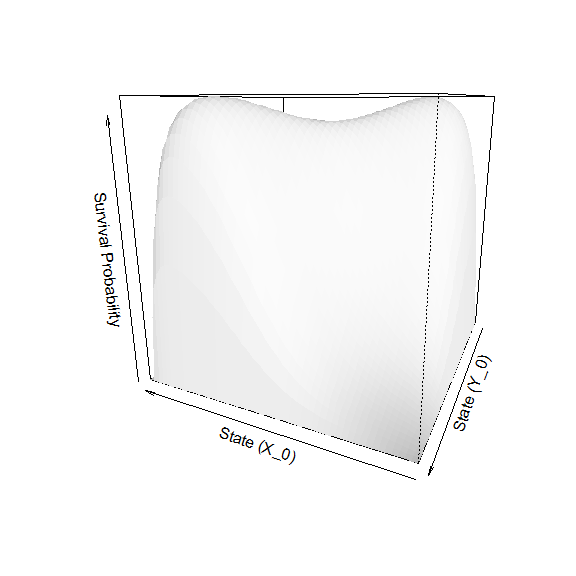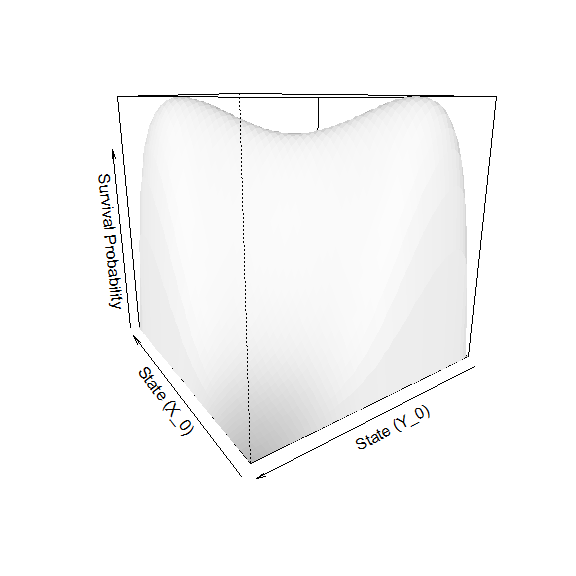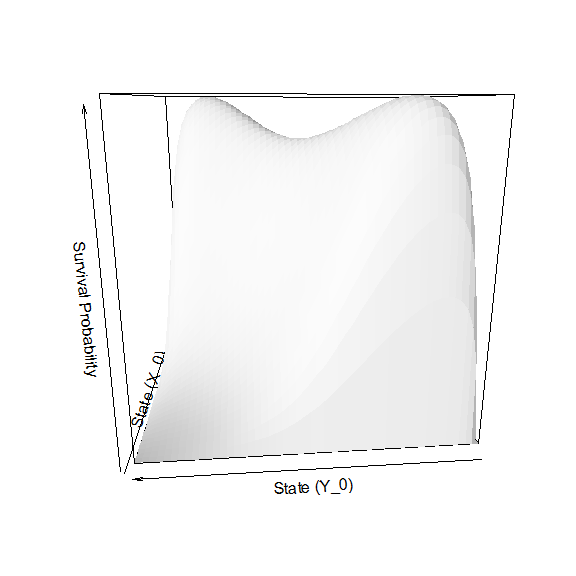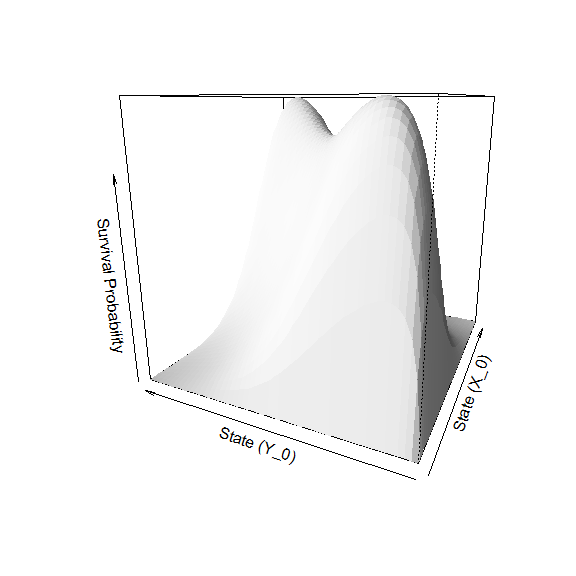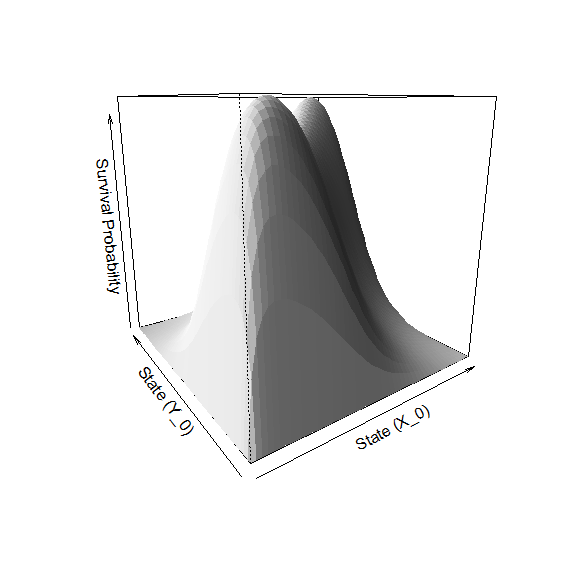plot(res$density~res$time, col = '#222299', type = 'l',
main = 'First passage time density')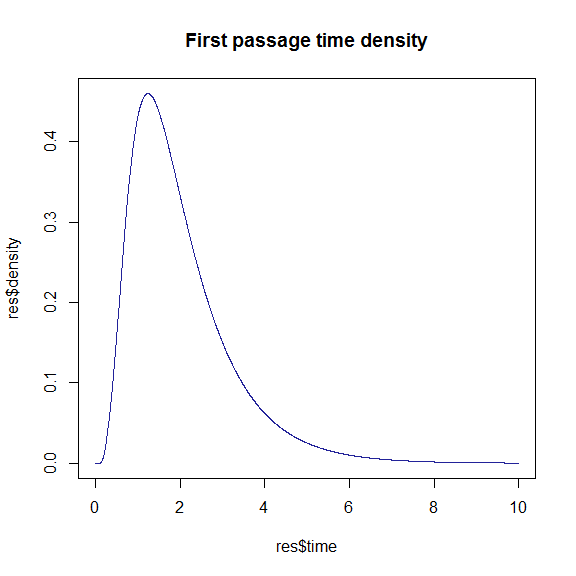#-------------------------------------------------------------------------------

## First escape from a non-square region

Consider a bivariate Ornstein-Uhlenbeck process:

\begin{aligned} dX_t &=[0.5X_t(1 -X_t^2)+Y_t]dt + dB_t^{(1)}\\ dY_t &=[0.5Y_t(1 -Y_t^2)-X_t]dt + dB_t^{(2)}.\\ \end{aligned}

We can approximate the survival probability surface for the process $$(X_t,Y_t)$$ escaping from a unit circle centred at $$(0.5,0.5)$$ by passing an indicator function to the BiMOL.passage() function. Here, the indicator function assigns points of the discretization lattice values of 0 or 1 depending on whether a given point on the lattice is within the region of interest or not. In R:

# Define drift and diffusion terms:
mu1   <- function(X,Y){-1*X}
mu2   <- function(X,Y){-1*Y}
sig11 <- function(X,Y){0.5}
sig22 <- function(X,Y){0.5}

Nodes <- 101
delt  <- 1/500

# Define a region in the x-y plane:
region <- function(x,y){sqrt((x-0.5)^2+(y-0.5)^2)<1}
res    <- BiMOL.passage(0.5, 0.5, 10, c(-1,2,-1,2), Nodes, delt, Phi = region)

# Draw a nice plot of the evolution of the survival prob.:
library("colorspace")
colpal=function(n){rev(sequential_hcl(n,power=0.8,l=c(40,100)))}
time.sequence <- c(0.1, 0.25, 0.5, 1)
k = 0
for(i in time.sequence)
{
k = k+1
filled.contour(res$Xt, res$Yt, res$surface[,,i/delt], color.palette = colpal, main = paste0('Survival probability \n (t = ', i,')'), xlab = expression(X), ylab = expression(Y), plot.axis = { th =seq(0,1,1/100) lines(0.5+sin(2*pi*th),0.5+cos(2*pi*th)) abline(h = 0, v = 0, lty = 'dashed') }) }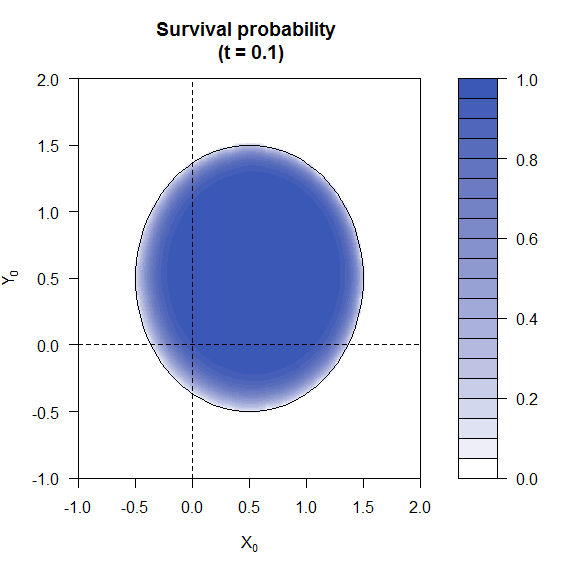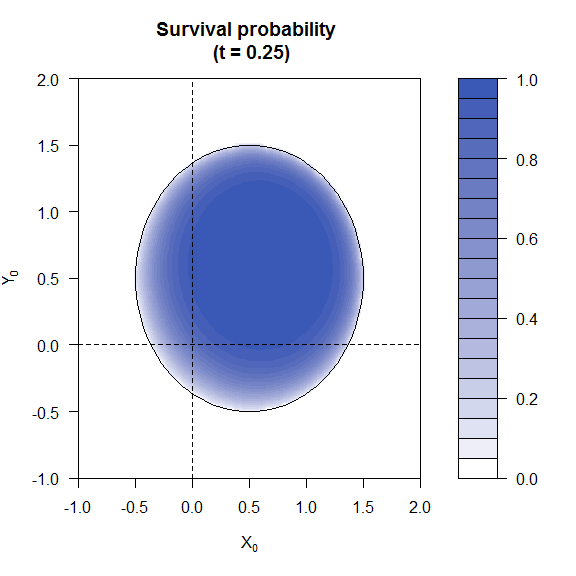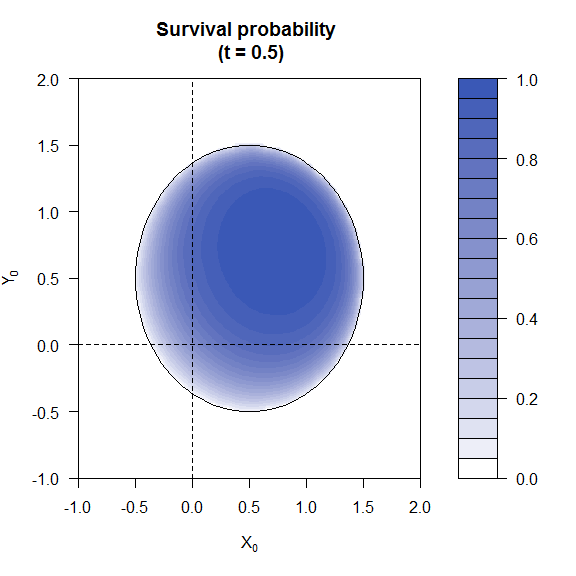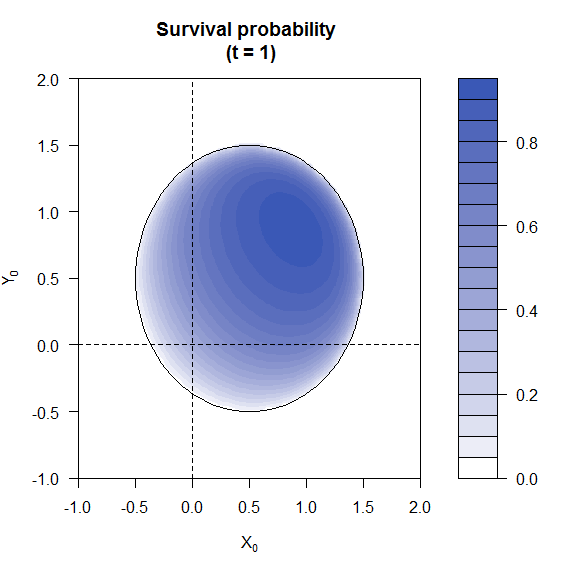We can compare this to simulated first passage times as follows… NOTE: The simulation algorithm used here will typically over-estimate first passage times. Consequently, the frequency distribution will be slightly off/biased (See Giraudo and Sacerdote (1999)). See for example the effect of changing the stepsize. sim = function(N = 50000, plt = FALSE) { set.seed(200707881) delt = 1/2000 times = rep(0, N) X = rep(0.5, N) Y = rep(0.5, N) k = 1 d = 0 while(N >1) { X = X + mu1(X,Y)*delt + sig11(X,Y)*rnorm(N,sd = sqrt(delt)) Y = Y + mu2(X,Y)*delt + sig22(X,Y)*rnorm(N,sd = sqrt(delt)) d = d + delt wh = (sqrt((X-0.5)^2+(Y-0.5)^2)>=1) if(plt) { plot(X,Y,pch = 20,xlim = c(-1,2), ylim =c(-1,2)) th =seq(0,1,1/100) lines(0.5+sin(2*pi*th),0.5+cos(2*pi*th)) } if(any(wh)) { X = X[which(!wh)] Y = Y[which(!wh)] m = N - length(X) N = length(X) times[k:(k+m-1)] = d k = k+m } } return(times) } sm = sim() hist(sm, freq = FALSE, border = 'white', col= 'gray75', ylim = range(res$density), xlim = c(0, 6))
lines(res$density~res$time, col = '#222299', lwd = 2)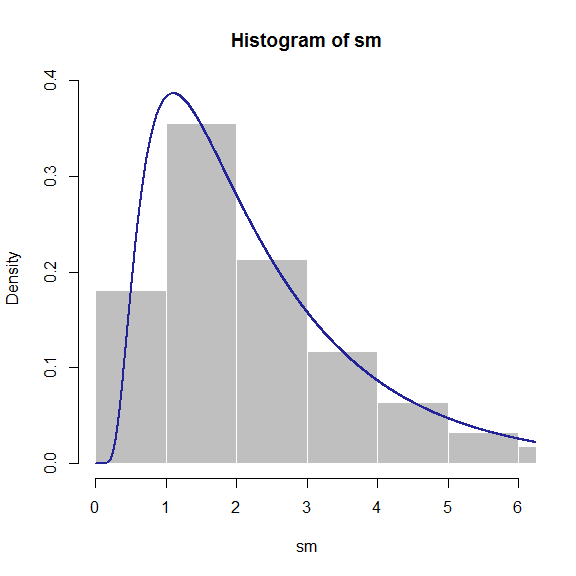To get a visualization of the problem, you can run a small scale simulation with a plot using the command sim(50, TRUE).

# Further reading

• Related packages:
• More Vignettes:
browseVignettes('DiffusionRimp')